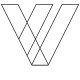Edges: Bar and Space Transitions

In 401

As we’ve said before, barcode scanners work based on reflectivity and contrast.

The messages encoded in linear barcodes are decoded on the basis of the scanner’s ability to detect reflective and contrast differences.

In a pattern of lines and spaces, these critical differences are demarked by the edges—the places where the bar ends and the space begins. We think of these as hard boundaries but in fact, with a little magnification, we can see that what we think of as a hard edge is really more like a transition.

Edges are more like a transition than a hard delineation

Edges actually have width–just like bars and spaces do. The smaller that transition or width, the better; but whether an edge has a wide transition or a narrow one, there will be a width.

Edges with wide transition makes it difficult for the scanner to determine where the bar terminates and the space begins, so edge determination is important enough to be one of the nine ANSI parameters.

Edges (Edge Determination) gauge how the scanner sees the edge between bars and spaces

Edge determination: measuring how a scanner is seeing edges. How can we see edges as scanners see them? The tool used by all ISO compliant verifiers is the Scan Reflectance Profile (SRP).

The SRP is a graphical representation of what the scanner “sees.” The upper half of the chart graphs the light reflectance features of the barcode—this is the quiet zones and the spaces between the bars. The top of the chart represents 100% reflectivity. The lower half graphs the dark reflectance features—the bars. The bottom of the chart represents 0% reflectivity.

The height of the graph is divided by an equatorial line called the Global Threshold or GT. It is at the GT where the scanner detects the reflectance and contrast transitions—this is where the edges are (or should be). The GT is where the scanner counts bars and spaces to determine what kind of symbol it is seeing.

A high performing barcode will product an SRP that is tall and symmetrical: the upper and lower halves of the graph will be equidistant from the GT; every bar and space will penetrate the GT and extend from it, up and down, equally.

The ANSI parameter called Edge Determination is what establishes where the GT line is located. Here is the formula:

GT = Reflectance minimum + (Symbol Contrast divided by 2)

Edges establish the location of the Global Threshold line

In the previous article, we learned that Reflectance minimum is the reflectance value of the bars, which must always be the dark elements; Symbol Contrast is a straight subtraction of the dark reflectance value from the light reflectance value.

The result of the calculation determines where the GT line is located for that particular symbol. Once the GT is established, transitions through the GT result in the bar/space count. If any bars or spaces fail to penetrate the GT, the symbol fails this parameter. This is almost always due to wide transition edges. Edge Determination is a Pass/Fail parameter. Failure here also results in failure of the Decode parameter.

Edges are a key factor in the whole verification process. It is possible for edges to pass this parameter and fail a subsequent parameter such as Reflectance Minimum or Edge Contrast Minimum, but if edges fail, nothing else matters.

Recent Posts
•Greg

What is the minimum spacing between the edge of a label to the start of a 1D Code 3 of 39 bar code?

•John Nachtrieb

Greg:

Code 39 quiet zones vary depending on the size of the X dimension (the width of the narrow bar). The algorithm for Code 39 quiet zones is greater than or equal to 10 times X.

A high quality ISO compliant verifier will automatically calculate this value and apply it to the verification grade.

Thank you for your comment.

•Greg

What is the minimum spacing between the edge of a label to the start of a 1D Code 3 of 39 bar code?

•John Nachtrieb

Greg:

Code 39 quiet zones vary depending on the size of the X dimension (the width of the narrow bar). The algorithm for Code 39 quiet zones is greater than or equal to 10 times X.

A high quality ISO compliant verifier will automatically calculate this value and apply it to the verification grade.

Thank you for your comment.